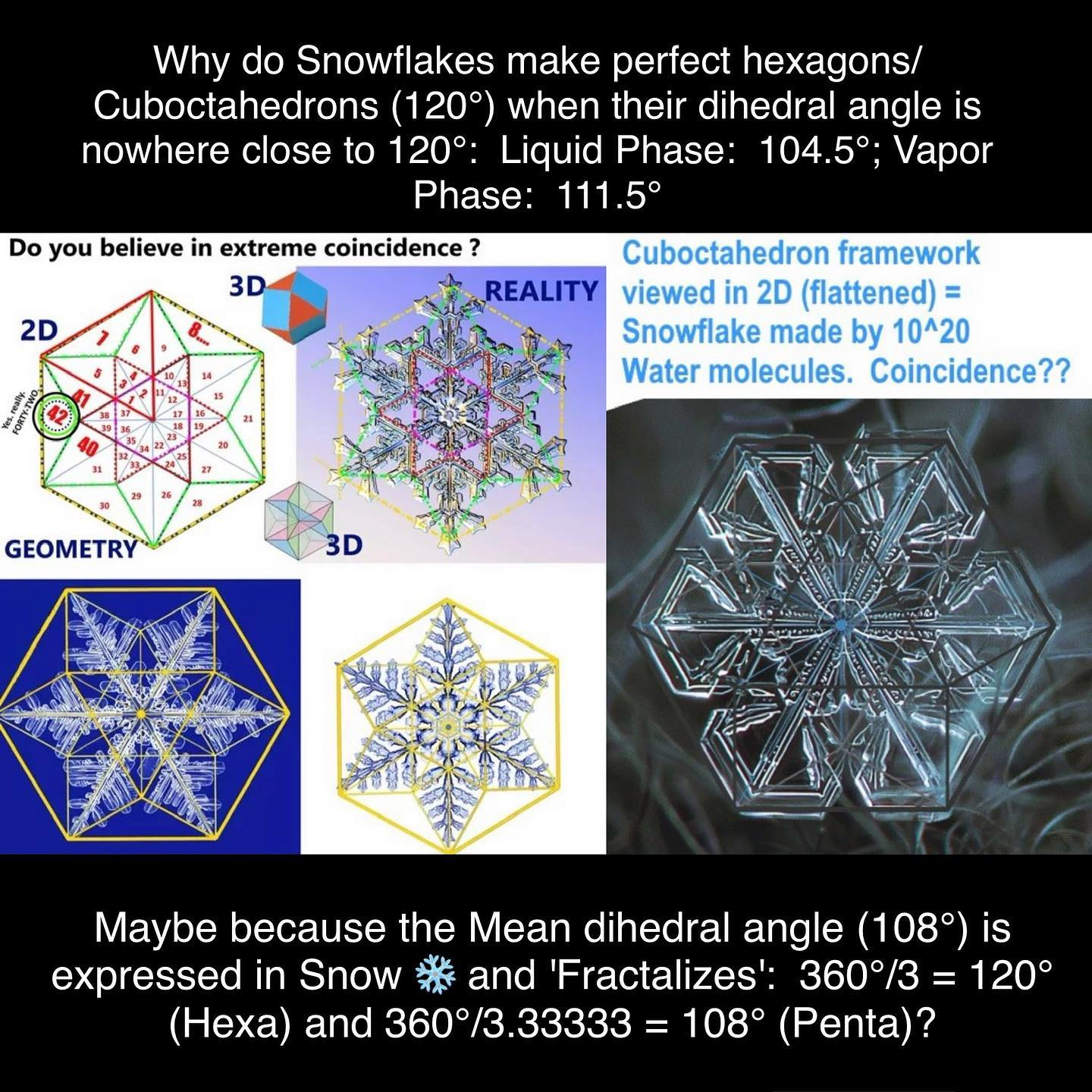I’ve been doing a lot of research work lately on potential (yet unknown) Phase Transitions (Beyond Liquid, Gas/Vapor and Solid/Ice) of Water and measurement of the respective Dihedral Angles of water molecules through these transition phases and what this might mean for the clustering Geometries.

The Dihedral angle of Snow (which is differentiated from ICE/Solid) is not presently confirmed/known leading to the mystery of why Snow takes the shape of perfect hexagons particularly when none of the various phase Dihedral angles are even remotely close to 120°.

An argument can be made for 108° Dihedral angles enabling hexagonal matrices as 360° / 3 = 120° and 360° / 3.3333… = 108°, where 3.33333 represents an infinitely mirrored fractal of the number 3. This phenomenon is also true for all other numbers like 6: 360° / 6 = 60° (Hexa angle) ; 360° / 6.6666… = 54° (Penta angle (54°*2 = 108°).

The Water Vapor Dihedral = 111.5°; the Water Liquid Dihedral = 104.5°. The Mean Angle = 108°.

One other consideration: as Water represents such a significant aspect of the superstructure of DNA 🧬, does the above inherency of Water toward BOTH the Hexagonal and the Pentagonal geometric associations point to potentially why the nucleotide pairs (Adenine+Thymine; Cytosine+Guanine) of DNA are precisely Hexagonal and Pentagonal geometries?

Further, what other implications might the greater understanding of Water’s various Dihedral Angles portend to understanding the different phases of Water. Moreover, in a broader sense, are the transition phases of molecules (such as Water for example), actually DEFINED (perhaps entirely) by the transitions in their geometric dihedral angles?

If there is indeed a Fourth (or more) Phase of Water as Water Researcher Gerald Pollack posits, are there more (yet unknown) dihedral angles of Water’s subtle transition phases and therefore more to understand regarding both Genotypic and Phenotypic Expression?

“Applied Geometry = Physics. Applied Physics = Chemistry. Applied Chemistry = Biology”